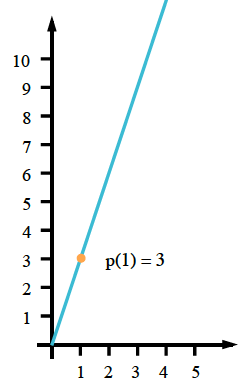### Home > A2C > Chapter 1 > Lesson 1.1.4 > Problem1-51

1-51.

Sketch a few different equilateral triangles. Create multiple representations ($x→y$ table, graph, equation) of the function with inputs that are the length of one side of an equilateral triangle and outputs that are its perimeter.

Start by creating some equilateral triangles of different sizes.

Use the triangles you drew to make a table.

Draw the graph.

Use the table of the graph to help you write the equation.

$\left. \begin{array} { | c | c | } \hline 1 & { 3 } \\ \hline 2 & { 6 } \\ \hline 3 & { 9 } \\ \hline 4 & { 12 } \\ \hline 5 & { 15 } \\ \hline 6 & { 18 } \\ \hline \end{array} \right.$Use the eTool below create multiple representations of the function.
Click the link at right for the full version of the eTool:  1-51 HW eTool